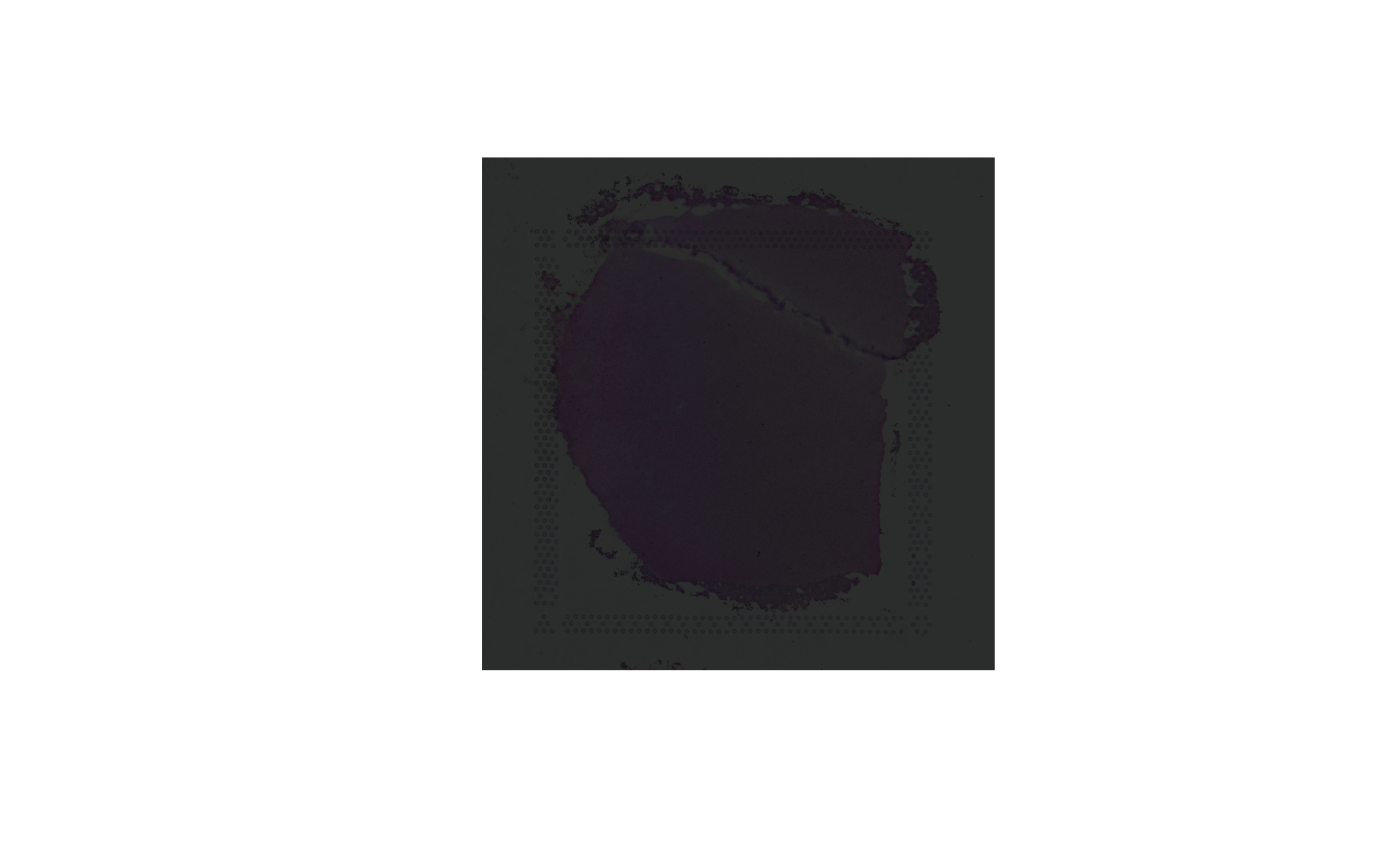This function uses the magick package to edit the color and perform other image manipulations on a background image. It can be useful if you want to highlight certain features of these images.

img_edit(
spe,
sampleid,
image_id = "lowres",
channel = NA,
brightness = 100,
saturation = 100,
hue = 100,
enhance = FALSE,
contrast_sharpen = NA,
quantize_max = NA,
quantize_dither = TRUE,
equalize = FALSE,
normalize = FALSE,
transparent_color = NA,
transparent_fuzz = 0,
background_color = NA,
negate = FALSE
)

## Arguments

spe

Defaults to the output of fetch_data(type = 'spe'). This is a SpatialExperiment-class object with the spot-level Visium data and information required for visualizing the histology. See fetch_data() for more details.

sampleid

A character(1) specifying which sample to plot from colData(spe)$sample_id (formerly colData(spe)$sample_name).

image_id

A character(1) with the name of the image ID you want to use in the background.

channel

A character(1) passed to magick::image_channel. If NA this step is skipped.

brightness

A numeric(1) passed to magick::image_modulate.

saturation

A numeric(1) passed to magick::image_modulate.

hue

A numeric(1) passed to magick::image_modulate.

enhance

A logical(1) controlling whether to use magick::enhance.

contrast_sharpen

A numeric(1) passed to magick::image_contrast. If NA this step is skipped.

quantize_max

A numeric(1) passed to magick::image_quantize. If NA this step is skipped.

quantize_dither

A logical(1) passed to magick::image_quantize.

equalize

A logical(1) controlling whether to use magick::equalize.

normalize

A logical(1) controlling whether to use magick::normalize.

transparent_color

A character(1) passed to magick::image_transparent. If NA this step is skipped.

transparent_fuzz

A numeric(1) passed to magick::image_transparent.

background_color

A character(1) passed to magick::image_background. If NA this step is skipped.

A numeric(1) passed to magick::image_median. If NA this step is skipped.

negate

A logical(1) controlling whether to use magick::negate.

## Value

A magick image object such as the one returned by magick::image_read.

## Details

The magick functions are used in the sequence represented by the arguments to this function. You can alternatively use this function sequentially. Or directly use the magick package.

Other Image editing functions: img_update_all(), img_update()

## Examples

if (enough_ram()) {
## Obtain the necessary data
if (!exists("spe")) spe <- fetch_data("spe")

## Reduce brightness to 25%
x <- img_edit(spe, sampleid = "151507", brightness = 25)
plot(x)
}